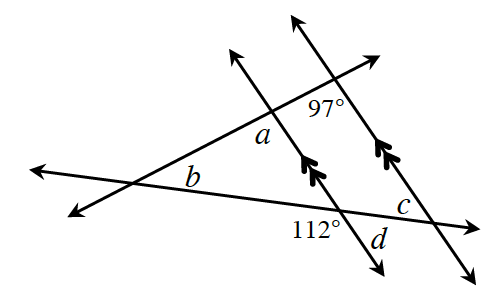### Home > CCG > Chapter Ch8 > Lesson 8.3.3 > Problem8-121

8-121.

Examine the relationships that exist in the diagram below. Find the measures of angles $a, b, c,$ and $d$. Remember that you can find the angles in any order, depending on the angle relationships you use.Refer to the Math Notes box from Lesson 2.1.4 for extra help.

Use the angles that you know to find the measures of those you do not know.
Use things like corresponding angles, linear pairs, alternate interior angles and the triangle sum theorem.